# Intro

This vignette is intended to provide several examples of different network visualization methods in the `ggplot2` / “tidyverse” framework. The methods, the `geomnet` package, the `ggnetwork` package, and the function `GGally::ggnet2`, are detailed in the paper Network Visualization with `ggplot2` by the authors of this vignette.

# Required Packages

``````library(dplyr)
library(tidyr)
library(ggplot2) # needs to be version ≥ 2.1.0
library(scales)

## ggnet2
if (!require(GGally, quietly = TRUE)) {
getFromNamespace("install_github", asNamespace("devtools"))("ggobi/ggally")
}``````
``````##
## Attaching package: 'GGally'``````
``````## The following object is masked from 'package:dplyr':
##
##     nasa``````
``````## geom_net
if (!require(geomnet, quietly = TRUE) ||
packageVersion("geomnet") < "0.2.0") {
getFromNamespace("install_github", asNamespace("devtools"))("sctyner/geomnet")
}

## ggnetwork
if (!require(ggnetwork, quietly = TRUE) ||
packageVersion("ggnetwork") < "0.5.1") {
getFromNamespace("install_github", asNamespace("devtools"))("briatte/ggnetwork")
}

library(network)``````
``````## network: Classes for Relational Data
## Version 1.13.0 created on 2015-08-31.
## copyright (c) 2005, Carter T. Butts, University of California-Irvine
##                     Mark S. Handcock, University of California -- Los Angeles
##                     David R. Hunter, Penn State University
##                     Martina Morris, University of Washington
##                     Skye Bender-deMoll, University of Washington
##  For citation information, type citation("network").
##  Type help("network-package") to get started.``````
``library(sna)``
``## Loading required package: statnet.common``
``````## sna: Tools for Social Network Analysis
## Version 2.4 created on 2016-07-23.
## copyright (c) 2005, Carter T. Butts, University of California-Irvine
##  For citation information, type citation("sna").
##  Type help(package="sna") to get started.``````
``````library(GGally)
library(geomnet)
library(ggnetwork)
library(igraph)``````
``````##
## Attaching package: 'igraph'``````
``````## The following objects are masked from 'package:sna':
##
##     betweenness, bonpow, closeness, components, degree,
##     dyad.census, evcent, hierarchy, is.connected, neighborhood,
``````## The following objects are masked from 'package:network':
##
##     delete.vertices, get.edge.attribute, get.edges,
##     get.vertex.attribute, is.bipartite, is.directed,
##     list.edge.attributes, list.vertex.attributes,
##     set.edge.attribute, set.vertex.attribute``````
``````## The following objects are masked from 'package:tidyr':
##
##     %>%, crossing``````
``````## The following objects are masked from 'package:dplyr':
##
##     %>%, as_data_frame, groups, union``````
``````## The following objects are masked from 'package:stats':
##
##     decompose, spectrum``````
``````## The following object is masked from 'package:base':
##
##     union``````

# Mad Men Relationship Network Example

## `geomnet`

``````# make data accessible

# code for geom_net
# data step: merge edges and nodes by the "from" column

``````## Using Name1 as the from node column and Name2 as the to node column.
## If this is not correct, rewrite dat so that the first 2 columns are from and to node, respectively.``````
``## Joining edge and node information by from_id and label respectively.``
``````# create plot
set.seed(10052016)
ggplot(data = MMnet, aes(from_id = from_id, to_id = to_id)) +
geom_net(aes(colour = Gender), layout.alg = "kamadakawai",
size = 2, labelon = TRUE, vjust = -0.6, ecolour = "grey60",
directed =FALSE, fontsize = 3, ealpha = 0.5) +
scale_colour_manual(values = c("#FF69B4", "#0099ff")) +
xlim(c(-0.05, 1.05)) +
theme_net() +
theme(legend.position = "bottom")``````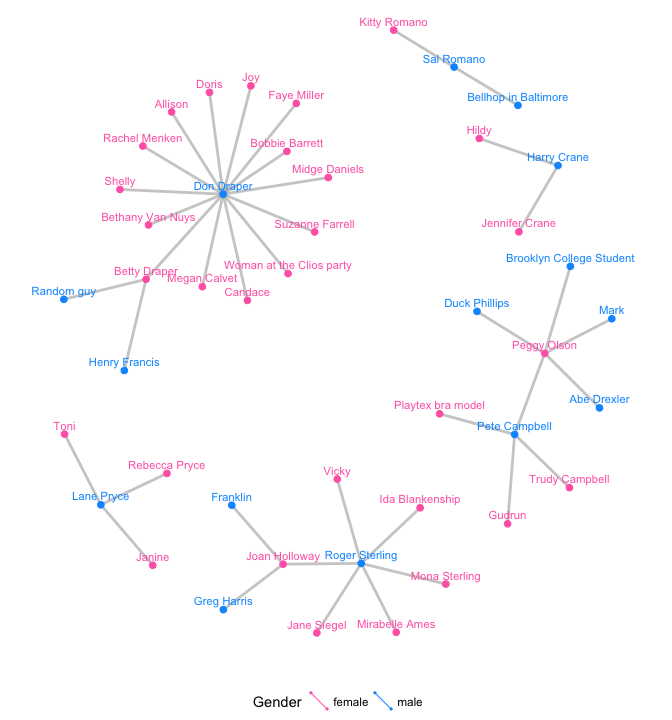Mad Men relationship network included in the `gcookbook` package by Winston Chang visualized using `geomnet`.

## `ggnet2`

`````` library(GGally)
library(network)
# make the data available
# data step for both ggnet2 and ggnetwork
# create undirected network
mm.net <- network::network(madmen\$edges[, 1:2], directed = FALSE)
# create node attribute (gender)
mm.net %v% "gender" <- as.character(
)
# gender color palette
mm.col <- c("female" = "#ff69b4", "male" = "#0099ff")
# create plot for ggnet2
set.seed(10052016)
ggnet2(mm.net, color = mm.col[ mm.net %v% "gender" ],
label = TRUE, label.color = mm.col[ mm.net %v% "gender" ],
size = 2, vjust = -0.6, mode = "kamadakawai", label.size = 3)``````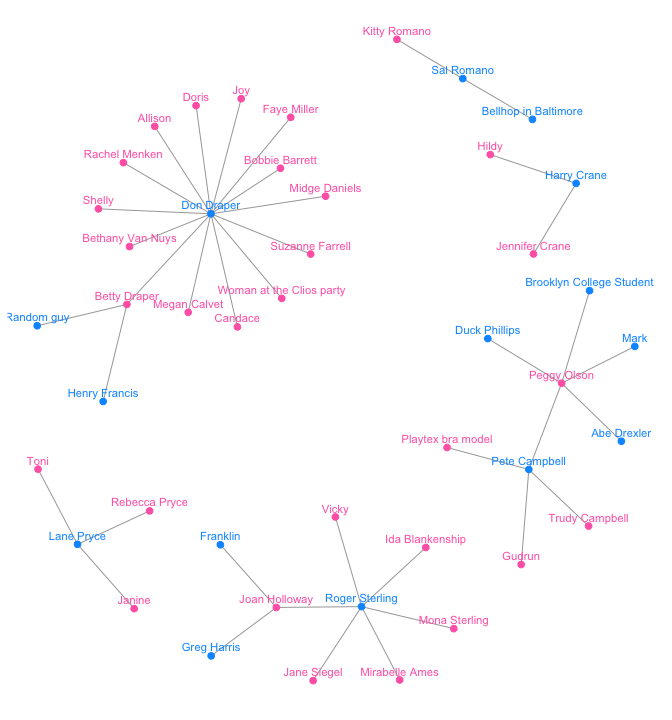Mad Men example using the `ggnet2` function in the `GGally` package.

## `ggnetwork`

``````# create plot for ggnetwork. uses same data created for ggnet2 function
library(ggnetwork)
set.seed(10052016)
ggplot(data = ggnetwork(mm.net, layout = "kamadakawai"),
aes(x, y, xend = xend, yend = yend)) +
geom_edges(color = "grey50") +
geom_nodes(aes(colour = gender), size = 2) +
geom_nodetext(aes(colour = gender, label = vertex.names),
size = 3, vjust = -0.6) +
scale_colour_manual(values = mm.col) +
xlim(c(-0.05, 1.05)) +
theme_blank() +
theme(legend.position = "bottom")``````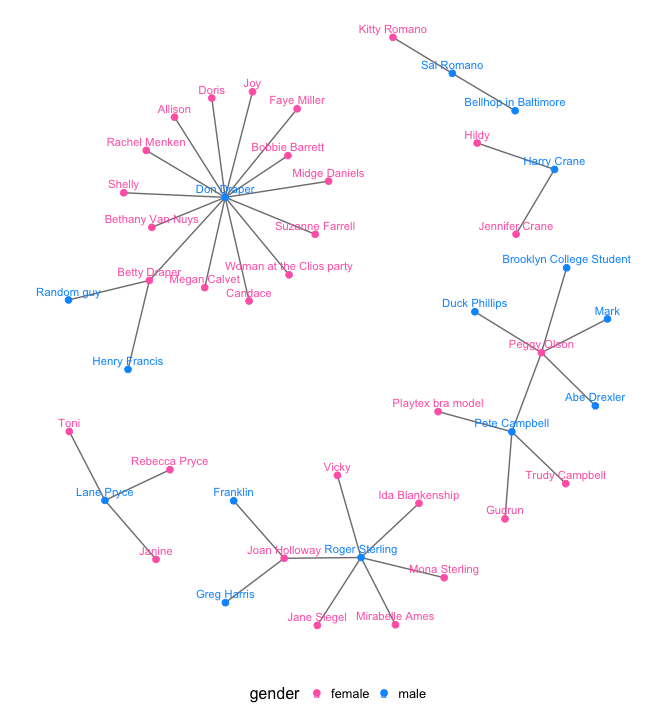Mad Men example using the `ggnetwork` package.

# Blood Donation Example

Blood donation “network”: which blood types can give and receive?

## `ggnet2`

``````# make data accessible
data(blood, package = "geomnet")

# plot with ggnet2 (Figure 2a)
set.seed(12252016)
ggnet2(network::network(blood\$edges[, 1:2], directed=TRUE),
mode = "circle", size = 15, label = TRUE,
arrow.size = 10, arrow.gap = 0.05, vjust = 0.5,
node.color = "darkred", label.color = "grey80")``````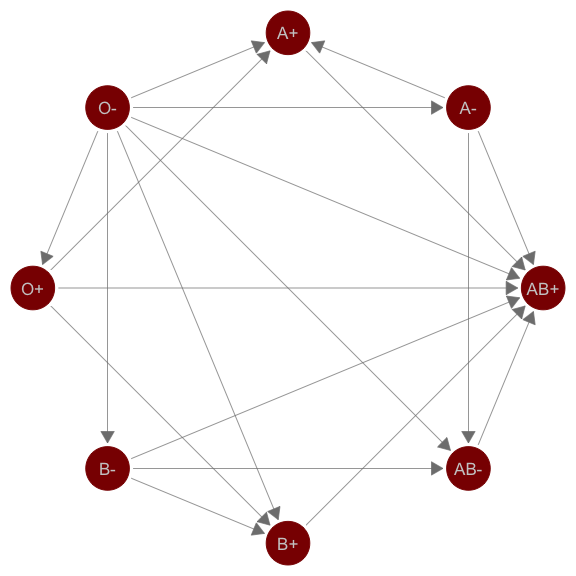`ggnet` implementation

## `geomnet`

``````# plot with geomnet (Figure 2b)
set.seed(12252016)
ggplot(data = blood\$edges, aes(from_id = from, to_id = to)) +
geom_net(colour = "darkred", layout.alg = "circle", labelon = TRUE, size = 15,
directed = TRUE, vjust = 0.5, labelcolour = "grey80",
arrowsize = 1.5, linewidth = 0.5, arrowgap = 0.05,
selfloops = TRUE, ecolour = "grey40") +
theme_net() ``````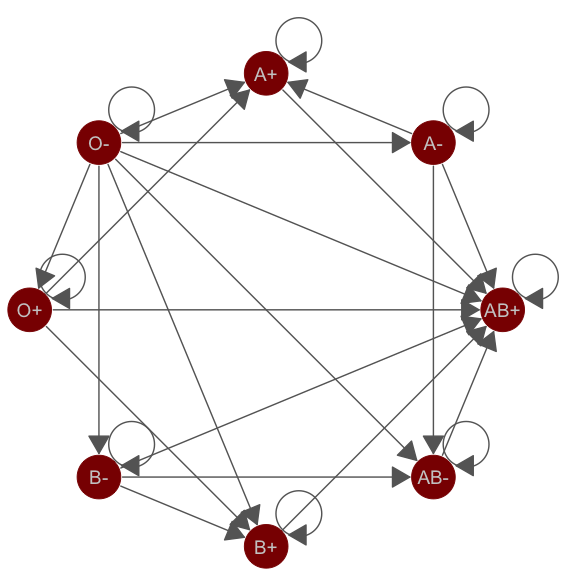`geom_net` implementation

## `ggnetwork`

``````# plot with ggnetwork (Figure 2c)
set.seed(12252016)
ggplot(ggnetwork(network::network(blood\$edges[, 1:2]),
layout = "circle", arrow.gap = 0.05),
aes(x, y, xend = xend, yend = yend)) +
geom_edges(color = "grey50",
arrow = arrow(length = unit(10, "pt"), type = "closed")) +
geom_nodes(size = 15, color = "darkred") +
geom_nodetext(aes(label = vertex.names), color = "grey80") +
theme_blank()``````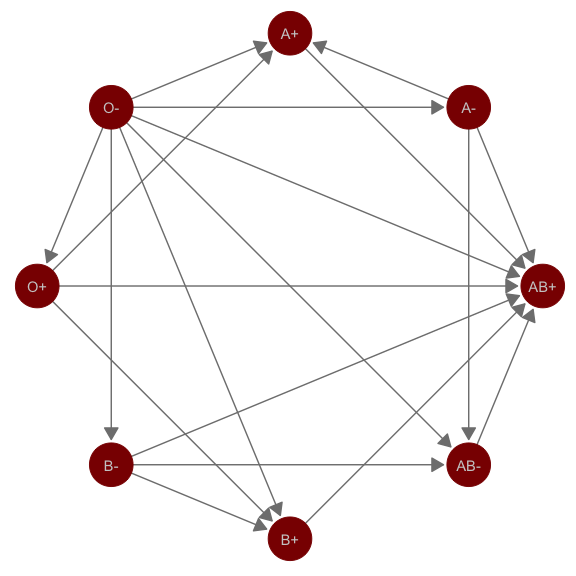`ggnetwork` implementation

# Email Network Example

A faux company’s email network provided by the 2014 VAST Challenge.

## `ggnet2`

``````# make data accessible
data(email, package = 'geomnet')

# create node attribute data
em.cet <- as.character(
email\$nodes\$CurrentEmploymentType)
names(em.cet) = email\$nodes\$label

# remove the emails sent to all employees
edges <- subset(email\$edges, nrecipients < 54)
# create network
em.net <- edges[, c("From", "to") ]
em.net <- network::network(em.net, directed = TRUE)
# create employee type node attribute
em.net %v% "curr_empl_type" <-
em.cet[ network.vertex.names(em.net) ]
set.seed(10312016)
ggnet2(em.net, color = "curr_empl_type",
size = 4, palette = "Set1",
arrow.size = 5, arrow.gap = 0.02,
edge.alpha = 0.25, mode = "fruchtermanreingold",
edge.color = c("color", "grey50"),
color.legend = "Employment Type") +
theme(legend.position = "bottom")``````
``## Warning: Removed 727 rows containing missing values (geom_segment).``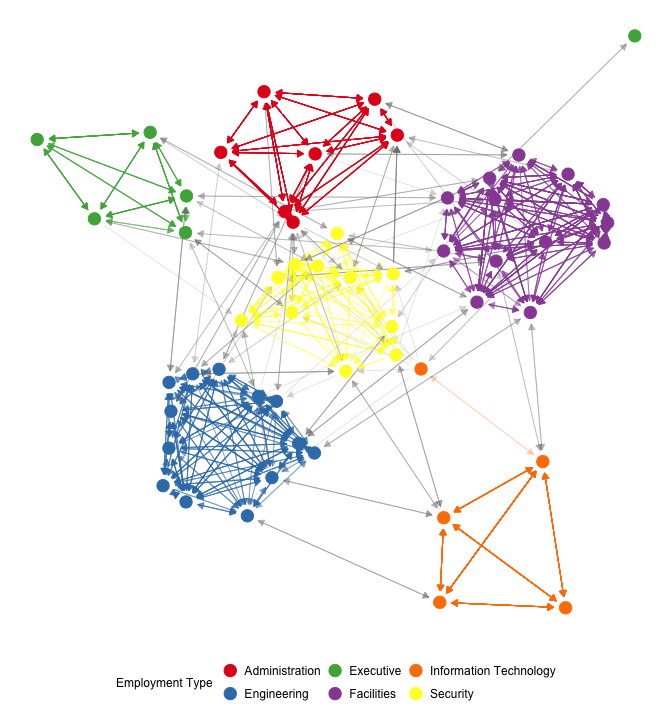The company’s email network visualized with `ggnet2`.

## `geomnet`

``````# data step for the geomnet plot
email\$edges <- email\$edges[, c(1,5,2:4,6:9)]
emailnet <- fortify(
as.edgedf(subset(email\$edges, nrecipients < 54)),
email\$nodes)``````
``````## Using From as the from node column and to as the to node column.
## If this is not correct, rewrite dat so that the first 2 columns are from and to node, respectively.``````
``## Joining edge and node information by from_id and label respectively.``
``````set.seed(10312016)
ggplot(data = emailnet,
aes(from_id = from_id, to_id = to_id)) +
geom_net(layout.alg = "fruchtermanreingold",
aes(colour = CurrentEmploymentType,
group = CurrentEmploymentType,
linewidth = 3 * (...samegroup.. / 8 + .125)),
ealpha = 0.25,
size = 4, curvature = 0.05,
directed = TRUE, arrowsize = 0.5) +
scale_colour_brewer("Employment Type", palette = "Set1") +
theme_net() +
theme(legend.position = "bottom")``````